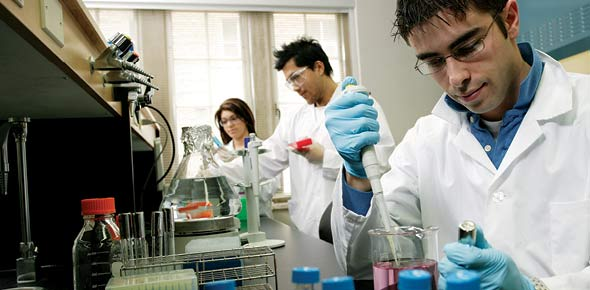# 10 Principles Of Bioenergetics

12 Questions | Attempts: 2471
ShareSettings• 1.
Describe why anabolism and catabolism need to be precisely regulated. Include in your answer what is meant by reciprocal regulation of anabolic and catabolic pathways.
• 2.
Describe using an equation, how a reaction in a biochemical pathway may occur spontaneously despite the standard free energy for that reaction being positive. Outline the difference between free energy and standard free energy in your answer.
• 3.
Which is INCORRECT?  Energy is required by cells for:
• A.

Transport of molecules or ions against a concentration gradient

• B.

Synthesis of new chemical bonds

• C.

Maintenance of body temperature

• D.

Mechanical movement

• E.

Hydrolysis of a molecule during an exothermic reaction

• 4.
Which statement about catabolic reactions is true?
• A.

Catabolic reactions are degradative and are generally exergonic

• B.

Catabolic reactions are part of pathways responsible for synthesis for new chemical bonds

• C.

Catabolic reactions generally have free enthalpy values close to zero and standard free energy values less than zero

• D.

Catabolic reactions occur primarily in catabolic organisms

• E.

Catabolic reactions oppose the second law of thermodynamics

• 5.
Which is NOT a mechanism for regulating metabolic pathways?
• A.

Control of intracellular substrate concentration

• B.

Control of allosteric enzymes by inhibitors and activators

• C.

Control of cell temperature

• D.

Control of amount of enzymes

• E.

Control of enzymes through signalling substances (e.g. hormones)

• 6.
Reciprocal regulation refers to:
• A.

The division of energy from the catalytic pathway by the anabolic pathway

• B.

The opposite reaction type that is being referred to

• C.

The ability of cells to simultaneously activate anabolic and catabolic reactions

• D.

The ability of cells to simultaneously inactive anabolic and catabolic reactions

• E.

Activation of one pathway, while suppressing the opposite pathway

• 7.
If the ΔG'° of the reactions A → B is +20kJ/mol, under standard conditions the reaction:
• A.

Will proceed spontaneously from right to left

• B.

Will proceed spontaneously from left to right

• C.

Will never reach equilbrium

• D.

Will proceed to equilibrium at a rapid rate

• E.

Is currently at equilibrium

• 8.
If the ΔG'° of the reaction A → B is -100 kJ/mol, under standard conditions the reaction:
• A.

Is at equilbirum

• B.

Will never reach equilibrium

• C.

Will not occur spontaneously

• D.

Will proceed at a rapid rate

• E.

Will proceed spontaneously from left to right

• 9.
Which is NOT true about the following reactions?A → B   ΔG = +20 kJ/mol = reaction 1C → D   ΔG = –30 kJ/mol = reaction 2 A + C → B + D = reaction 3
• A.

Reaction 1 will proceed from right to left

• B.

Reaction 2 will proceed from left to right

• C.

Reaction 3, the sum of reaction 1 and 2, will occur spontaneously with overall free energy of –10 kJ/mol

• D.

Reaction 1 will not proceed from left to right unless an enzyme is present

• E.

Reaction 2 will proceed spontaneously

• 10.
Given the following information, calculate the actual free energy of the below reaction. A → B;  Keq = 10; concentration in the cell of A = 0.2 M; B = 0.05 M; R = 8.315 J/mol.K; T = 298 K?
• A.

-9.1 kJ/mol

• B.

-15.8 kJ/mol

• C.

-200.2 kJ/mol

• D.

+22.5 kJ/mol

• E.

0 kJ/mol

• 11.
When a mixture containing an equal concentration of glucose 6-phosphate and fructose 6-phosphate is incubated with the enzyme phosphohexose isomerase, the final mixture contains twice as much glucose 6-phosphate as fructose 6-phosphate.  Which one of the following statements is correct, when applied to the reaction below (R = 8.315 J/mol•K and T = 298 K)? Glucose 6-phosphate ↔ fructose 6-phosphate
• A.

ΔG'° is +1.7 kJ/mol

• B.

ΔG'° is –1.7 kJ/mol

• C.

ΔG'° is incalculably large and negative

• D.

ΔG'° is incalculably large and positive

• E.

ΔG'° is zero

• 12.
What is the standard-state free energy (ΔG°) for the hydrolysis of ATP to ADP?
• A.

+45.6 kJ/mol

• B.

-45.6 kJ/mol

• C.

-30.5 kJ/mol

• D.

15.6 kJ/mol

• E.

12.2 kJ/mol

## Related TopicsBack to top
×

Wait!
Here's an interesting quiz for you.